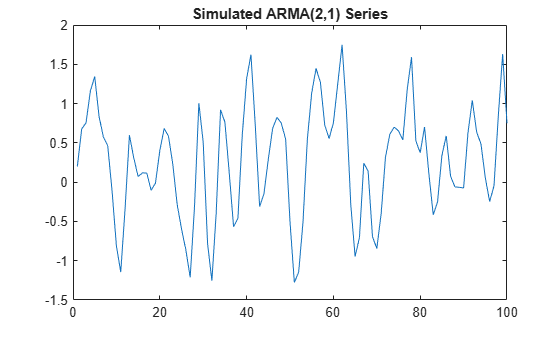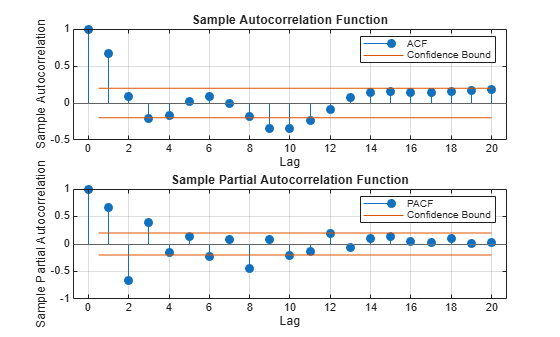Documentation

## Choose ARMA Lags Using BIC

This example shows how to use the Bayesian information criterion (BIC) to select the degrees p and q of an ARMA model. Estimate several models with different p and q values. For each estimated model, output the loglikelihood objective function value. Input the loglikelihood value to `aicbic` to calculate the BIC measure of fit (which penalizes for complexity).

### Step 1. Simulate an ARMA time series.

Simulate an ARMA(2,1) time series with 100 observations.

```modSim = arima('Constant',0.2,'AR',{0.75,-0.4},... 'MA',0.7,'Variance',0.1); rng('default') Y = simulate(modSim,100); figure plot(Y) xlim([0,100]) title('Simulated ARMA(2,1) Series')```### Step 2: Plot the sample ACF and PACF.

Plot the sample autocorrelation function (ACF) and partial autocorrelation function (PACF) for the simulated data.

```figure subplot(2,1,1) autocorr(Y) subplot(2,1,2) parcorr(Y)```Both the sample ACF and PACF decay relatively slowly. This is consistent with an ARMA model. The ARMA lags cannot be selected solely by looking at the ACF and PACF, but it seems no more than four AR or MA terms are needed.

### Step 3. Fit ARMA(p,q) models.

To identify the best lags, fit several models with different lag choices. Here, fit all combinations of p = 1,...,4 and q = 1,...,4 (a total of 16 models). Store the loglikelihood objective function and number of coefficients for each fitted model.

```LOGL = zeros(4,4); % Initialize PQ = zeros(4,4); for p = 1:4 for q = 1:4 mod = arima(p,0,q); [fit,~,logL] = estimate(mod,Y,'Display','off'); LOGL(p,q) = logL; PQ(p,q) = p+q; end end```

### Step 4: Calculate the BIC.

Calculate the BIC for each fitted model. The number of parameters in a model is p + q + 1 (for the AR and MA coefficients, and constant term). The number of observations in the data set is 100.

```LOGL = reshape(LOGL,16,1); PQ = reshape(PQ,16,1); [~,bic] = aicbic(LOGL,PQ+1,100); reshape(bic,4,4)```
```ans = 4×4 108.6241 105.9489 109.4164 113.8443 99.1639 101.5886 105.5203 109.4348 102.9094 106.0305 107.6489 99.6794 107.4045 100.7072 102.5746 102.0209 ```

In the output BIC matrix, the rows correspond to the AR degree (p) and the columns correspond to the MA degree (q). The smallest value is best.

The smallest BIC value is `99.1639` in the (2,1) position. This corresponds to an ARMA(2,1) model, matching the model that generated the data.